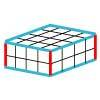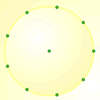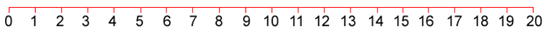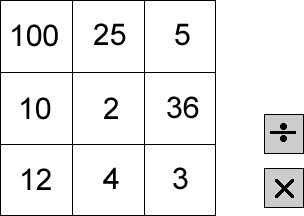#### You may also like### Round and Round the Circle

What happens if you join every second point on this circle? How about every third point? Try with different steps and see if you can predict what will happen.### Making Cuboids

Let's say you can only use two different lengths - 2 units and 4 units. Using just these 2 lengths as the edges how many different cuboids can you make?How many DIFFERENT quadrilaterals can be made by joining the dots on the 8-point circle?

# Four Go

##### Age 7 to 11Challenge Level

Draw a number line on a piece of paper, marked from $0$ to $20$, like this:(You could print off this sheet which has several number lines drawn on it.)

This challenge is a game for two players.  The first player chooses two numbers in this grid and either multiplies or divides them.He or she then marks the answer to the calculation on the number line.The second player then chooses two numbers and either $\times$ or $\div$, and marks that number in a different colour on the number line.

If the answer is too big or too small to be marked on the number line, the player misses a go.

The winner is the player to get four marks in a row with none of their opponent's marks in between.

What good ways do you have of winning the game?

Does it matter if you go first or second?

[This game is adapted from a SMILE Centre card.]